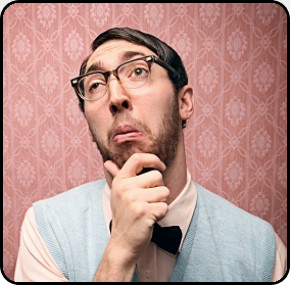Related Questions

 Tags

Question by  gshort6228aolcom (30)

# In numerology, how do I determine what is my number?

 +1 vote! +7 you voted Answer by  Lillian (87) Use the numeral of the day of the month of your birthday. If you were born on the 15th, add 1 + 5, for a core number of 6.

 +1 vote! +7 you voted Answer by  brainstorm (20) Your number is the day of the month that you were born. If you were born on a day that has a double digit, then add the two numbers.

 +1 vote! +4 you voted Answer by  anamaradancer (114) YOu use your name and birth date to figure out the numbers and use those to determine your number. Chinese Numerology is different. Wikipedia has a good page on Numerology.

 +1 vote! +4 you voted Answer by  ashleydoll (93) add all the numbers in your birth date together: November 19, 1987 would be 11/19/1987 or 11 + 19 + 19 + 87 = 147. Then add those digits: 1 + 4 +7 = 11. Eleven is the only number in which you don't add the digits together to get a single number

 +1 vote! +2 you voted Answer by  Michelle54 (13) Your number is determined by the day you were born. If your birthdate double digits, such as the 23, you would simply add the 2 and 3.

 add your own answer here You have 50 words left!